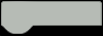I tried to control the robot arm with image recognition of HuskyLens.

### Face recognition of HuskyLensHuskyLens's Face Recognition is capable of learning and identifying multiple faces.

The method of multiple face recognition is as follows.
https://wiki.dfrobot.com/HUSKYLENS_V1.0_SKU_SEN0305_SEN0336#target_15

By default, the face recognition function can only learn and identify a specific face. Press and hold the Function button and select Learn Multiple to turn it ON and save.This method makes it possible to learn and identify multiple faces.Learning is done by pointing the camera and pressing the Learning button when the face is recognized and the ID is given.

Press the Learning button again to enter the next face recognition mode. In the same way, recognize another face and press the Learning button to impersonate the ID.

Learned and recognized three faces (ID1, ID2, ID3).I tried to move the robot arm by deriving the angle from the three center coordinates of this face recognition.

### Constitution

The center coordinates of the three faces recognized by HuskyLens are serially transmitted to Arduino.Move the servo at the angle calculated from 3 points with Arduino.Since software serial and servo control could not coexist,I am using hardware serial (D0, D1).### Robot ArmI use one axis of the robot arm made earlier.

### Angle Calculation

The angle is calculated from the center coordinates of the three faces recognized by HuskyLens.Each side of the three-point triangle isAngle from cosine theorem Is calculated by### Arduino code

I programmed using the official Husky Lens Arduino tutorial.Tutorial: Arduino Tutorial

Arduino library: https://github.com/HuskyLens/HUSKYLENSArduino

Reference sample code: HUSKYLENS_GET_STARED.ino

CODE
``````#include "HUSKYLENS.h"
#include "SoftwareSerial.h"
#include <Servo.h>

Servo myservo;
HUSKYLENS huskylens;

int x1, y1, x2, y2, x3, y3;
float a, b, c, th;

void setup() {
Serial.begin(9600);
myservo.attach(6, 820, 2140); //DS3115

while (!huskylens.begin(Serial)){
Serial.println(F("Begin failed!"));
Serial.println(F("1.Please recheck the \"Protocol Type\" in HUSKYLENS (General Settings>>Protocol Type>>Serial 9600)"));
delay(100);
}
}

void loop() {
if (!huskylens.request()) {}
else if(!huskylens.isLearned()) {}
else if(!huskylens.available()) {}
else{
while (huskylens.available()){
if (result.command == COMMAND_RETURN_BLOCK){
if(result.ID == 1){
x1 = result.xCenter;
y1 = result.yCenter;
} else if(result.ID == 2){
x2 = result.xCenter;
y2 = result.yCenter;
}else if(result.ID == 3){
x3 = result.xCenter;
y3 = result.yCenter;
}
}

a = sqrt(float((x1 -x3)*(x1 -x3)) + float((y1 -y3)*(y1 -y3)));
b = sqrt(float((x1 -x2)*(x1 -x2)) + float((y1 -y2)*(y1 -y2)));
c = sqrt(float((x2 -x3)*(x2 -x3)) + float((y2 -y3)*(y2 -y3)));

th=acos((b*b + c*c - a*a)/(2*b*c)) * 180.0/M_PI ;
myservo.write(int(th));
delay(100);
}
}
}``````

### Operation

The servo motor of the robot arm is operated by recognizing the three faces and calculating the angle.